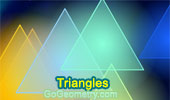# Triangles: Theorems and Problems 25Proposed Problem 186. Right Triangle, Altitude, Incenters, Circles.

Proposed Problem 185. Trapezoid, Triangles and Angles.

Proposed Problem 184. Triangle and Angles.

Proposed Problem 183. Right Triangle, Hypotenuse Trisection Points, Squares of the Distances.

Proposed Problem 180. Circles Tangent Externally, Common External Tangents, Areas.

Proposed Problem 179. Rhombus, Square, Diagonal, Angle.

Proposed Problem 174. Quadrilateral with Midpoints, Triangles, Areas.

Proposed Problem 173. Quadrilateral with Midpoints, Triangles, Areas.

Proposed Problem 172. Trapezoid, Midpoints, Quadrilaterals, Areas.

Proposed Problem 171. Trapezoid, Midpoints, Triangles, Areas.

Proposed Problem 170. Trapezoid, Midpoint, Triangle, Area.

Proposed Problem 169. Parallelogram, Interior and Exterior Points, Diagonals, Pentagon, Triangles, Areas

Proposed Problem 168. Parallelogram, Diagonal, Triangles, Pentagon, Areas.

Go to Page: Previous | 1 | 2 | 3 | 4 | 5 | 6 | 7 | 8 | 9 | 10 | 11 | 12 | 13 | 14 | 15 | 16 | 17 | 18 | 19 | 20 | 21 | 22 | 23 | 24 | 25 | 26 | 27 | 28 | 29 | 30 | 40 | Next

 Home | Search | Geometry | Polygon | Email | By Antonio Gutierrez